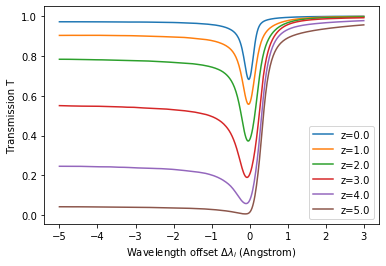Make sure to have enough bandwidth and disk space (~9 GB).

In :
import requests
import shutil
import sys

fn = "lyaigmcurves.hdf5"
with requests.get(url, stream=True) as r:
with open(fn, 'wb') as f:
dl = 0
for data in r.iter_content(chunk_size=2**24):
dl += len(data)
f.write(data)
d = int(32*dl/ltot)
sys.stdout.write("\r Progress: [%s%s]" % ("="*d, " "*(32-d)) )
sys.stdout.flush()

 Progress: [================================]

# Take a look at the data¶

## Data Structure¶

The data is given as HDF5 data set in the structure described here. We use the h5py python wrapper to read the data.

In :
import h5py
"""Load result of computation from hdf5 file."""
with h5py.File(f, "r") as h5file:
try:
return h5file[key][:]
except ValueError:
return h5file[key][()] # scalars


We have the following data available that are loaded and described further down:

In :
with h5py.File(fn,"r") as hf:
print(hf.keys())

<KeysViewHDF5 ['dlambda_bins', 'haloIDs', 'los_vectors', 'tau']>


## Meta data¶

### Redshifts¶

We provide data for various redshift snapshots:

In :
with h5py.File(fn,"r") as hf:
print(hf["tau"].keys())

<KeysViewHDF5 ['z0.0', 'z1.0', 'z2.0', 'z3.0', 'z4.0', 'z5.0']>


### Wavelengths bins¶

The optical depths are computed for input wavelengths $\Delta_i$ of Ly$\alpha$ photons injected in the halo center of the respective emitter evaluated at the emitters' redshift. For convenience, we express the wavelength as difference to the Ly$\alpha$ line-center wavelength $\lambda_c$: $\Delta\lambda_i = \lambda_i-\lambda_c$. Units in Angstrom.

In :
dlambdas = load_result(fn,"dlambda_bins")
print("We have %i wavelength bins equally spaced from %.1f to %.1f Angstrom around the line-center."%(dlambdas.shape,dlambdas,dlambdas[-1]))

We have 401 wavelength bins equally spaced from -5.0 to 3.0 Angstrom around the line-center.


### Lines of Sight¶

In this catalog, we provide 6 lines of sight for each emitter roughly aligned with the coordinate axes.

In :
los_vecs = load_result(fn,"los_vectors")
los_vecs

Out:
array([[ 1.000000e+00, -1.168596e-16,  4.965581e-18],
[ 3.532461e-02,  9.977882e-01, -5.631044e-02],
[ 6.187621e-02,  1.011606e-02,  9.980326e-01],
[-9.994750e-01,  1.267281e-02,  2.981817e-02],
[ 2.383625e-02, -9.989999e-01, -3.782849e-02],
[ 3.277323e-02,  3.418858e-02, -9.988779e-01]])

### Halo IDs¶

Each emitter is associated with an IllustrisTNG100 halo id. At each redshift, we provide the halo IDs for IllustrisTNG, so that the respective transmission curves can be associated back to a halo - for example to check for correlations with halo properties. Only star-forming halos were considered.

In :
hids = {}
for z in range(6):
hids

Out:
{'z0.0': array([   0,    1,    1, ..., 1716, 1828, 1888]),
'z1.0': array([     0,      1,      1, ..., 108145, 108207, 110065]),
'z2.0': array([     0,      1,      2, ..., 159251, 159823, 161240]),
'z3.0': array([     0,      1,      2, ..., 147720, 147751, 149709]),
'z4.0': array([     0,      1,      2, ..., 119842, 120200, 122121]),
'z5.0': array([    0,     1,     2, ..., 81561, 82060, 82324])}

## Optical Depths¶

The hdf5 group "tau" contains the optical depths as a data set for the respective redshift. For example, we can easily plot the mean transmission curves:

In :
import numpy as np
import matplotlib.pyplot as plt
for z in range(0,6):
plt.xlabel(r"Wavelength offset $\Delta\lambda_i$ (Angstrom)")